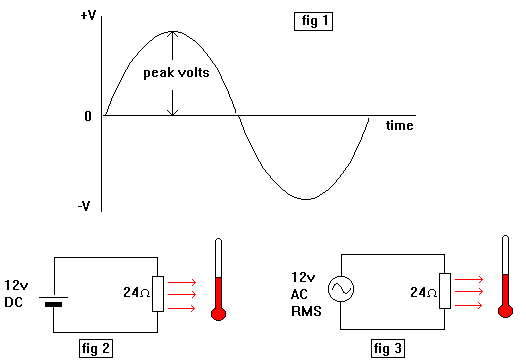Home > Electronic Tutorials > General Theory > RMS and Peak to Peak Tutorial

General Theory - Electronic Tutorials

# RMS and Peak to Peak Tutorial

If someone measures the value of the AC voltage coming out of a transformer using an oscilloscope and says it is 20 volts peak to peak and we use a voltmeter to confirm this we will find that the meter reads only 7.07 volts.

This is because the scope measures peak to peak values and the meter measures RMS values.

In figure 1 the 'scope displays the peak value. The peak to peak voltage is twice this. For example if the peak is 10 volts then the peak to peak is 20 volts.

When using a meter to measure the same AC voltage a different value is obtained. This is because, as we said, meters measure RMS values.

A Root Mean Square (RMS) voltage gives the same heating effect as a DC voltage of the same value.  See figures 2 and 3. Both thermometers show the same temperature when the resistors are heated by the current passing through them.

RMS values can be converted to peak to peak values and vice-versa.

RMS values times 1.414 equals the Peak value. Peak to Peak is twice this. 7.07 volts RMS times 1.414 and then doubled is 20 volts, the Peak to Peak value.Peak values times 0.707 gives the RMS value. Don't forget that Peak is half the Peak to Peak.

20 volts Peak to Peak is 10 volts Peak.

10 volts Peak times 0.707 equals 7.07 volts RMS.

Note: To report broken links or to submit your projects please send email to Webmaster

 Discover